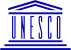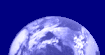Bilko Mini-lessons

# Mini-Lesson 4: Simple GIS exercise using Bilko

### Lesson Aims:

To introduce you to how a stacked set of raster layers in Bilko can be manipulated as a simple Geographic Information System (GIS) using formula documents to perform the necessary operations.

### Objectives:

By the end of this mini-lesson you will have carried out a simple GIS exercise to identify which parts of a 2.5 km x 2.5 km area of forest near a lake are suitable for logging, using a range of criteria. This nvolves a series of reclassification and Boolean GIS operations performed using formulas as well as use of one special Bilko GIS function that allows you to find the minimum distance between pixels and an identified feature.### Content Overview

This mini-lesson introduces you to the Geographical Information System functionality that is available in Bilko by applying formulas to stacks of raster layers. The mini-lesson also introduces a special GIS function to create a Euclidean Distance Transform (EDT) image.

You are provided with three data layers. In each data layer the value of the cells (or pixels) indicates an attribute for that position.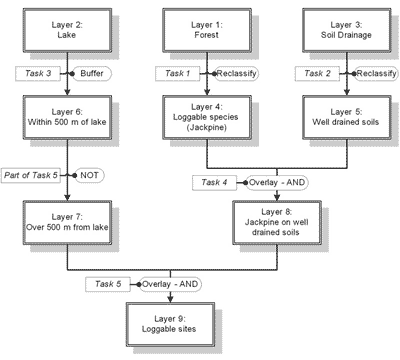Flow diagram showing a breakdown of the tasks required to identify commercially loggable sites based on the given criteria. Click here for larger version.

Layer 1: Forest - shows which forest tree species occur where. 1 = Black Spruce, 2 = Jackpine, 0 = No forest.

Layer 2: Lake - shows where waterbodies (in this case a lake) are located. 1 = lake, 0 = not lake.

Layer 3: Soil drainage - shows state of soil drainage. 0 = waterlogged, 1 = poor drainage, 2 = good drainage.

The purpose of the exercise is to identify areas suitable for logging. An area is suitable if it satisfies the following criteria:

(1) it is planted with Jackpine (Pinus banksiana), (2)

(2) it is well drained, and

(3) it is not within 500 m of a lake or watercourse.

### Sample images:

A selection of images from this mini lesson are shown below.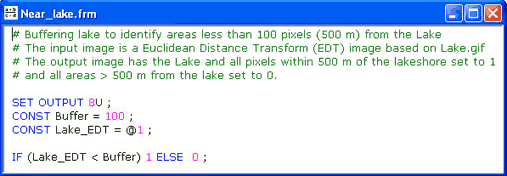Formula to identify the parts of the area that lie within 500 m (100 pixels) of the Lake and thus are not suitable for logging. This formula creates a buffer around the lake using a Euclidean Distance Transform layer where each non-lake pixel has a value equal to its distance in pixels (5 x 5 m) from the lake edge. Combined with the Euclidean Distance Transform option, this formula performs Task 3 in the flow diagram.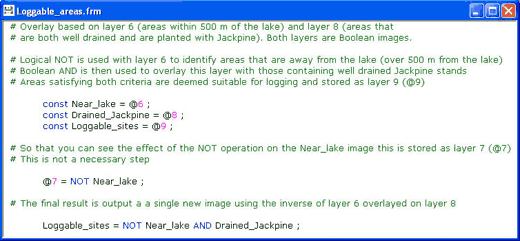Final formula to identify those areas in the image that are Jackpine on well-drained soils and more than 500 m from the lake. This formula performs Task 5 in the flow diagram.Result of Euclidean Distance Transform on the Lake (red=0). Each non-Lake cell now has a value equal to its distance from Lake in pixels.The Near_lake.frm formula document identifies areas (green=1) that lie within 500 m (100 pixel buffer zone) of the lake.Area of the image (green=1) that contains the loggable species (Jackpine).The actual area (green=1) of Jackpine that is suitable for logging according to the three criteria.Last update: 31 January 2018